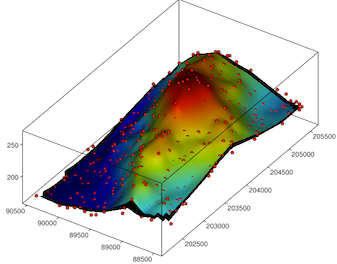• 本文主要演示如何使用matplotlib绘制图形。直接上代码，关键语句配有注释方便理解。import matplotlib as mplfrom mpl_toolkits.mplot3d import Axes3Dimport numpy as npimport matplotlib.pyplot as plt# 设置...
本文主要演示如何使用matplotlib绘制三维图形。直接上代码，关键语句配有注释方便理解。
import matplotlib as mpl
from mpl_toolkits.mplot3d import Axes3D
import numpy as np
import matplotlib.pyplot as plt
# 设置图例字号
mpl.rcParams['legend.fontsize'] = 10
fig = plt.figure()
# 设置三维图形模式
ax = fig.gca(projection='3d')
# 测试数据
theta = np.linspace(-4 * np.pi, 4 * np.pi, 100)
z = np.linspace(-4, 4, 100) / 4
r = z**3 + 1
x = r * np.sin(theta)
y = r * np.cos(theta)
# 绘制图形
ax.plot(x, y, z, label='parametric curve')
# 显示图例
ax.legend()
# 显示图形
plt.show()
运行结果：
展开全文• 本文实例讲述了Python使用matplotlib绘制参数曲线操作。分享给大家供大家参考，具体如下：一 代码import matplotlib as mplfrom mpl_toolkits.mplot3d importAxes3Dimport numpy as npimport matplotlib.pyplot ...
本文实例讲述了Python使用matplotlib绘制三维参数曲线操作。分享给大家供大家参考，具体如下：
一 代码
import matplotlib as mpl
from mpl_toolkits.mplot3d importAxes3D
import numpy as np
import matplotlib.pyplot as plt
mpl.rcParams['legend.fontsize']=10#图例字号
fig = plt.figure()
ax = fig.gca(projection='3d')#三维图形
theta = np.linspace(-4* np.pi,4* np.pi,100)
z = np.linspace(-4,4,100)*0.3#测试数据
r = z**3+1
x = r * np.sin(theta)
y = r * np.cos(theta)
ax.plot(x, y, z, label='parametric curve')
ax.legend()
plt.show()
二 运行结果希望本文所述对大家Python程序设计有所帮助。
展开全文• python绘制图 mplot3d tutorial官网 其他问题 绘制的三图如何旋转？ 其实.py脚本运行后的图本身就可以鼠标拖动旋转，但如果在 jupyter 中则需要加上一行代码（注意在VSCode内置的Jupyter中似乎无效） %...
基本绘制步骤
网上文章已经较多，写的比较好的
python绘制三维图mplot3d tutorial官网
其他问题
绘制的三维图如何旋转？
其实.py脚本运行后的图本身就可以鼠标拖动旋转，但如果在 jupyter 中则需要加上一行代码（注意在VSCode内置的Jupyter中似乎无效）
%matplotlib notebook

复数 j 是什么意思

mgrid[[1:3:3j, 4:5:2j]]

3j：3个点
步长为复数表示点数，左闭右闭步长为实数表示间隔，左闭右开
如何设置视角
详见文章 python 用 matplotlib 在 3D 空间绘制散点，体验不同视角 实例详解
# 调整视角
ax.view_init(elev=20,    # 仰角
azim=45    # 方位角
)

cmap有哪些可供选择？
这个参数目前见到的有两种设置方式，可选参数如下# 字符形式
cmap = 'spring'  # summer,autumn,winter 亦可
cmap = 'gist_earth'
# 调用 cm
from matplotlib import cm
cmap = cm.coolwarm
cmap = cm.rainbow

网格设置meshgrid和mgrid函数
详见文章 Python的 numpy中 meshgrid 和 mgrid 的区别和使用
如何使画出的曲面更光滑？
目前找到的解决方式只是通过griddata插值加密网格，关于griddata函数的用法可以参考
python 三维散点插值 griddata利用griddata进行二维插值
但这些例子基本都比较简单，基本都是单点或者几个点插值，如果是网格插值还需要进行一定的处理。下面给出一个示例代码
import  pandas  as pd
import  numpy as np
from scipy.interpolate import griddata

from mpl_toolkits.mplot3d import Axes3D
import matplotlib.pyplot as plt
from matplotlib import cm

'''
提取表格数据
'''
index0 = np.where(alpha==0)
alpha  = alpha[index0:]                #作图只选取[0,180]范围，不包括负攻角

Cl_df = Cl_df.interpolate(method='cubic')     #插值补全空缺值
Cl    = Cl_df.values[index0:,:]               #选择alpha在[0,180]范围的部分

'''
制作网格
'''
alpha_i = np.linspace(alpha.min(),alpha.max(),(len(alpha)*4))  #增加网格密度
Ma_i    = np.linspace(Ma.min(),   Ma.max(),   (len(Ma)*4))
Ma_i,alpha_i = np.meshgrid(Ma_i,alpha_i)

Ma, alpha = np.meshgrid(Ma, alpha)

'''
在加密网格上插值
'''
Cl_i = griddata((Ma.flatten(),  alpha.flatten()  ), Cl.flatten(),
(Ma_i.flatten(),alpha_i.flatten()), method='cubic')
Cl_i = Cl_i.reshape(np.shape(Ma_i))   # Ma_i和alpha_i的shape相同

'''
作图
'''
# CL绘图
fig = plt.figure(1)
surf = ax.plot_surface(Ma_i, alpha_i, Cl_i,cmap=cm.coolwarm,alpha=0.9)

# 调整视角
ax.view_init(elev=20,azim=-45)   # 默认仰角-60,方位角30
print(f'ax.azim {ax.azim}')
print(f'ax.elev {ax.elev}')

ax.contourf(Ma,alpha,Cl,zdir='x',offset=0,cmap=cm.coolwarm)    #等高线选择未加密网格数据
ax.contour(Ma,alpha,Cl,zdir='y',offset=190,cmap=cm.coolwarm)
ax.contour(Ma,alpha,Cl,zdir='z',offset=-20,cmap=cm.coolwarm)

plt.show()

但如果想要画出像下面这样的图还比较困难展开全文• 不过，我们仍然可以绘制出多维空间，今天就来用 Python 的 plotly 库绘制下三到六的图，看看长什么样。数据我们使用一份来自 UCI 的 真实汽车数据集 ，该数据集包括 205 个样本和 26 个特征，从中选择 6 个特征...
实用技巧。
我们的大脑通常最多能感知三维空间，超过三维就很难想象了。尽管是三维，理解起来也很费劲，所以大多数情况下都使用二维平面。不过，我们仍然可以绘制出多维空间，今天就来用 Python 的 plotly 库绘制下三维到六维的图，看看长什么样。
数据我们使用一份来自 UCI 的 真实汽车数据集 ，该数据集包括 205 个样本和 26 个特征，从中选择 6 个特征来绘制图形：基础工作
安装好 plotly 包：
pip install plotly
加载数据集（文末会提供）：
import pandas as pd
下面我们先绘制基础的二维图表，使用两个 RPM 和 Speed 两个特征即可：
绘制 2-D 图代码实现如下：
import plotly
import plotly.graph_objs as go
#绘制散点图
fig1 = go.Scatter(x=data['curb-weight'],
y=data['price'],
mode='markers')
#绘制布局
mylayout = go.Layout(xaxis=dict(title="curb-weight"),
yaxis=dict( title="price"))
#绘图 html
plotly.offline.plot({"data": [fig1],
"layout": mylayout},
auto_open=True)
保存为 html 文件打开可以生成交互界面，也可以保存为 png 图片。
下面增加特征来绘制三维图。
绘制 3-D 图
可以 使用 plotly 的 plot.Scatter3D 方法绘制三维图：代码实现如下：
fig1 = go.Scatter3d(x=data['curb-weight'],
y=data['horsepower'],
z=data['price'],
marker=dict(opacity=0.9,
reversescale=True,
colorscale='Blues',
size=5),
line=dict (width=0.02),
mode='markers')
mylayout = go.Layout(scene=dict(xaxis=dict( title="curb-weight"),
yaxis=dict( title="horsepower"),
zaxis=dict(title="price")),)
plotly.offline.plot({"data": [fig1],
"layout": mylayout},
auto_open=True,
filename=("3DPlot.html"))
如何绘制更高维度的图呢？显然无法通过扩展坐标轴的形式，不过有个小技巧就是制造一个虚拟维度，可以用不同颜色、形状大小、形状类别来入手。这样就可以显示第四个维度了。
绘制 4-D 图
下面我们将第四个变量——车辆油耗（city-mpg）添加到原先的三维图中，用颜色深浅表示，这样就绘制出了四维图。可以看到当其他三个指标（马力、车身重量、车价格）越高时：车辆油耗是越少的。绘制 5-D 图
基于这样的思想，我们还可以通过修改圆形大小再增加一个维度——发动机尺寸（engine-size）变成五维图：我们仍然可以比较容易地地发现：车越贵，发动机尺寸越大这样的规律。
绘制 6-D 图
接着还可以通过更改形状的方式增加第六个维度——车门数，圆形表示四车门，方形表示两车门。通常两个车门的都是昂贵的豪华跑车，在图中也可以看出方形主要集中在价格比较高的区域。这样我们就从普通的二维图扩展到了高维图，当然还可以继续拓展，不过分辨起来会越来越困难。
文中代码可以在后台回复： 6D 得到。
展开全文• 1. python图表绘制方法简介python图表的绘制算是二图表的一个进阶版本，本质上和二图表的绘制并无差别，唯一的区别在于使用的库略有差异。相较于二图表使用的pyplot库，三图表的绘制使用的是Axes3D...
• 1. python图表绘制方法简介python图表的绘制算是二图表的一个进阶版本，本质上和二图表的绘制并无差别，唯一的区别在于使用的库略有差异。相较于二图表使用的pyplot库，三图表的绘制使用的是Axes3D...
• 我就废话不多说了，直接上代码吧！ import matplotlib.pyplot as plt #也可以使用 import pylab ...x = [1,2,3,4,5,6] data1 = [1,1.3,1.39,1.41,1.42,1.40] data2 = [1,1.36,1.55,1.70,1.78,1.82] data3 = [1,1.6,2.
• Matplotlib库通常用来绘制曲面图形，下面我们给出了二曲线的一个标记的例子和一个绘制子图的例子；其余的例子都是绘制图形，这需要将坐标轴设置为三的坐标系，我们主要采用下列方式进行设置： fig = plt...
• 通常我们用 Python 绘制的都是二平面图，但有时也需要绘制场景图，比如像下面这样的： 这些图怎么做出来呢？今天就来分享下如何一步步绘制出三矢量（SVG）图。 八面体 我们先以下面这个八面体为例。 ...
• ## python绘制三维图

万次阅读 2019-02-15 14:46:41
作者：桂。 时间：2017-04-27 23:24:55 链接：http://www.cnblogs.com/xingshansi/p/6777945.html    本文仅仅梳理最基本的绘图方法。 一、初始化 假设已经安装了matplotlib工具包。... 4 ...
• ylabel('Y') ax.set_zlabel('Z') B-子图Subplot用法 与MATLAB不同的是，如果一个四子图效果，如： MATLAB： subplot(2,2,1) subplot(2,2,2) subplot(2,2,[3,4]) Python: subplot(2,2,1) subplot(2,2,2) subplot(2,1,...
• 通常我们用 Python 绘制的都是二平面图，但有时也需要绘制场景图，比如像下面这样的： 这些图怎么做出来呢？今天就来分享下如何一步步绘制出三矢量（SVG）图。 八面体 我们先以下面这个八面体为例。 ...
• 不过，我们仍然可以绘制出多维空间，今天就来用 Python 的 plotly 库绘制下三到六的图，看看长什么样。 数据我们使用一份来自UCI的真实汽车数据集，该数据集包括 205 个样本和 26 个特征，从中选择 6 个特征来...互联网 程序员
• 今天使用了python绘制图像的二切片，把Y固定为某一个值绘制X-Z切片，但是绘制出来的结果图发现X轴和Z轴发生了倒转，大概是这样： 左图为实际得到的不理想结果，右图为笔者想得到的结果： 2.矩阵和绘图规则 ...矩阵
• Matplotlib 也可以绘制 3D 图像，与二图像不同的是，绘制图像主要通过 mplot3d 模块实现。但是，使用 Matplotlib 绘制图像实际上是在二画布上展示，所以一般绘制图像时，同样需要载入 pyplot 模块。...
• 1. 绘制3D曲面图from matplotlib import pyplot as pltimport numpy as npfrom mpl_toolkits.mplot3d import Axes3Dfig=plt.figure()ax=Axes3D(fig)x=np.arange(-4,4,0.25)y=np.arange(-4,4,0.25)x,y=np.meshgrid(x,...
• 原标题：Python中使用Matplotlib绘制3D图形示例3D图形能给我们对数据带来更加深入地理解。python的matplotlib库就包含了丰富的3D绘图工具。3D图形在数据分析、数据建模、图形和图像处理等领域中都有着广泛的应用，...
• 不过，我们仍然可以绘制出多维空间，今天就来用 Python 的 plotly 库绘制下三到六的图，看看长什么样。 数据我们使用一份来自 UCI 的 真实汽车数据集 ，该数据集包括 205 个样本和 26 个特征，从中选择 6 个...
• Python绘制柱状图并在柱状图顶端添加标签 原创--Aurora-- 最后发布于2019-04-01 17:09:54 阅读数 889 收藏 展开 对于在Python中绘制三柱状图网上有很多的资料可以查询到了，笔者这边就不多赘述，直接上代码了...
• 今天使用python画了几个好玩的3D展示图，现在分享给大家。先贴上图片使用的python工具包为：from matplotlib import pyplot as plt import numpy as np from mpl_toolkits.mplot3d import Axes3D在...输入数据是三图片
• import matplotlib.pyplot as plt #也可以使用 import pylab as pl import matplotlib.font_manager as fm myfont = fm.FontProperties(fname=r'C:\Windows\Fonts\simkai.ttf') #或许字体，...x = [1,2,3,4,5,6] data1
• 使用Python绘制出类似Excel或者MATLAB的曲线还是比较容易就能够实现的，需要用到的额外库有两个，numpy和matplotlib。使用这两个模块实现的曲线绘制其实在一定程度上更像是MATLAB的plot功能，不过今天看了一下...
• python画图很多是根据z=f(x,y)来画图的，本博文将三个对应的坐标点输入画图：散点图：import matplotlib.pyplot as pltfrom mpl_toolkits.mplot3d import Axes3Dfig = plt.figure()ax = fig.add_subplot(111, ...
• ylabel('Y') ax.set_zlabel('Z') B-子图Subplot用法 与MATLAB不同的是，如果一个四子图效果，如： MATLAB： subplot(2,2,1) subplot(2,2,2) subplot(2,2,[3,4]) Python: subplot(2,2,1) subplot(2,2,2) subplot(2,1,...
• 经常遇到对所绘制图片进行标注的情况，下面进行一个简单的示例： #!/usr/bin/env python # # -*- coding: utf-8 -*- # # Author：'ZML' # # Time:2020/03/13 22:13 import matplotlib.pyplot as plt fig = ......

# python绘制4维python 订阅